# Circumference of a circle

Circumference of a circle
Go back to  'Circles'

 1 What is a Circle? 2 What is the Circumference of a Circle? 3 How to find the Circumference of a Circle? 4 Formula for the Circumference of a Circle 5 Important notes on Circumference of a Circle 6 Solved examples on Circumference of a Circle 7 Challenging Question on Circumference of a Circle 8 Practice Questions on Circumference of a Circle 9 Maths Olympiad Sample Papers 10 Frequently Asked Questions (FAQs)

We at Cuemath believe that Math is a life skill. Our Math Experts focus on the “Why” behind the “What.” Students can explore from a huge range of interactive worksheets, visuals, simulations, practice tests, and more to understand a concept in depth.

Book a FREE trial class today! and experience Cuemath's LIVE Online Class with your child.

## What is a Circle?

A Circle is a round closed figure where all its boundary points are equidistant from a fixed point called the centre.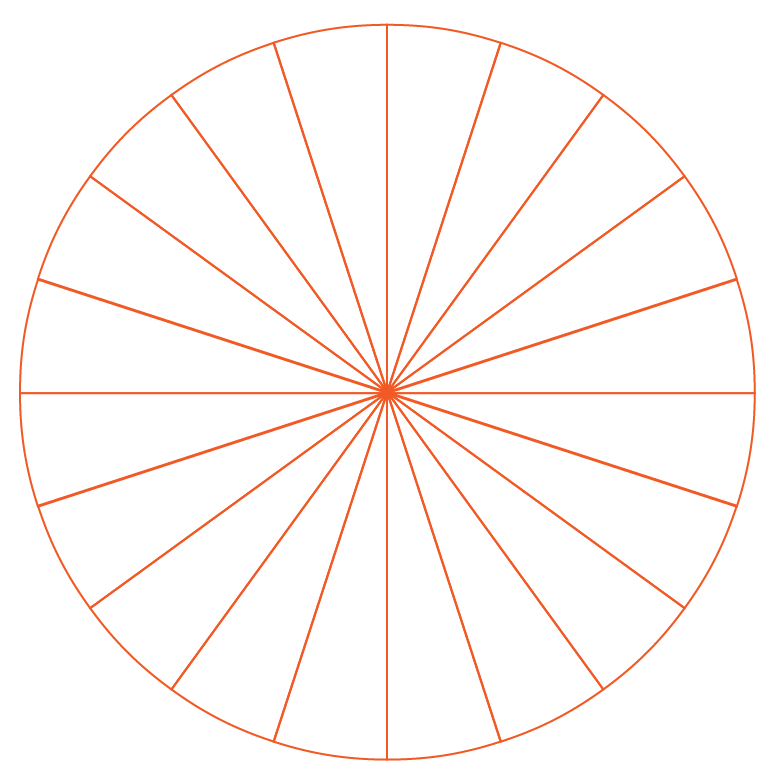Try this simulation to observe how a circle is formed.

Click on the buttons RESET and SEE.

You can observe that the collection of points are all equidistant from the centre.

## What is the Circumference of a Circle?

The circumference of a circle is its boundary or the length of the complete arc of a circle.

Let us understand this concept using an example.

Consider a circular park as shown below.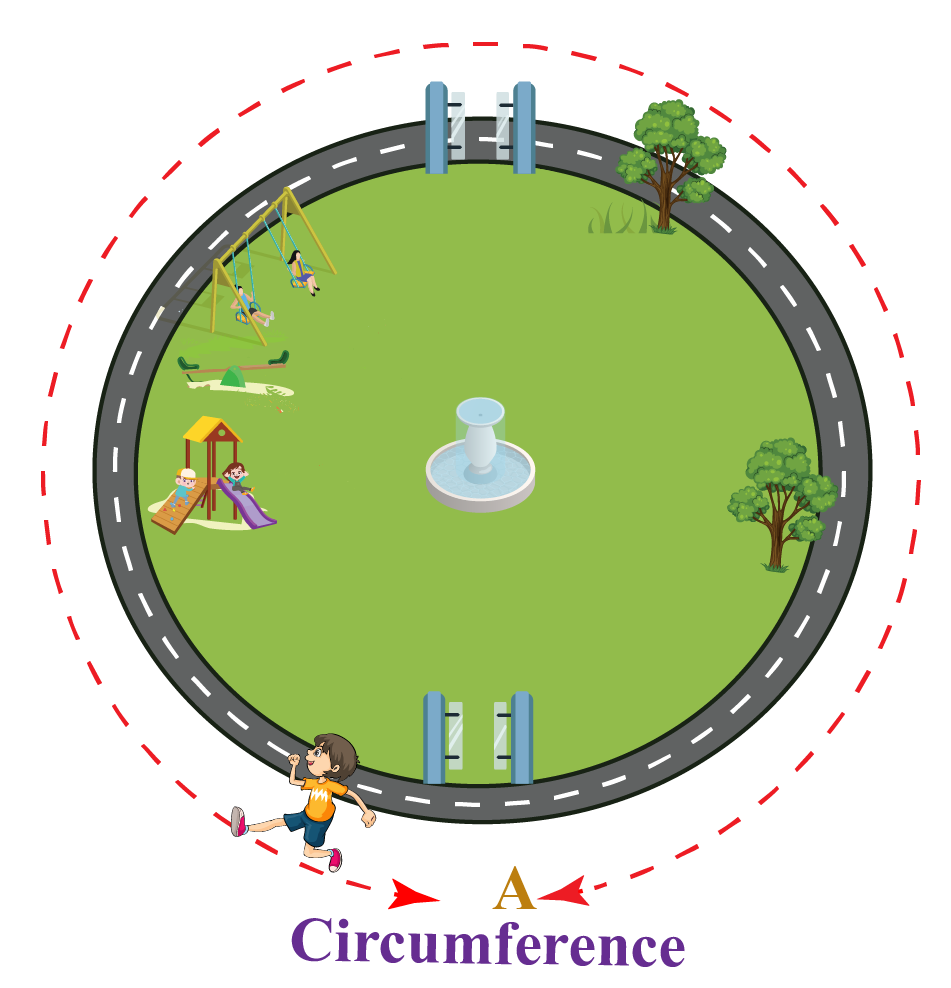If a boy starts running from point 'A' and reaches the same point after taking one complete round of the park, a distance is covered by him.

This distance or boundary is called the circumference of the park which is in the shape of a circle.

### Circumference is the Length of the Boundary

If we open a circle and measure the boundary just like we measure a straight line, we get the circumference of the circle in terms of units of length like centimetres, metres, or kilometres.

Click on "Go!" to observe the circumference measurements.

## How to Find the Circumference of a Circle?

Although the circumference of a circle is its length, it cannot be calculated with the help of a ruler (scale) like it is usually done for other polygons.

This is because a circle is a curved figure.

Therefore, to calculate the circumference of a circle, we apply a formula which uses the radius or the diameter of the circle and the value of Pi ($$\pi$$).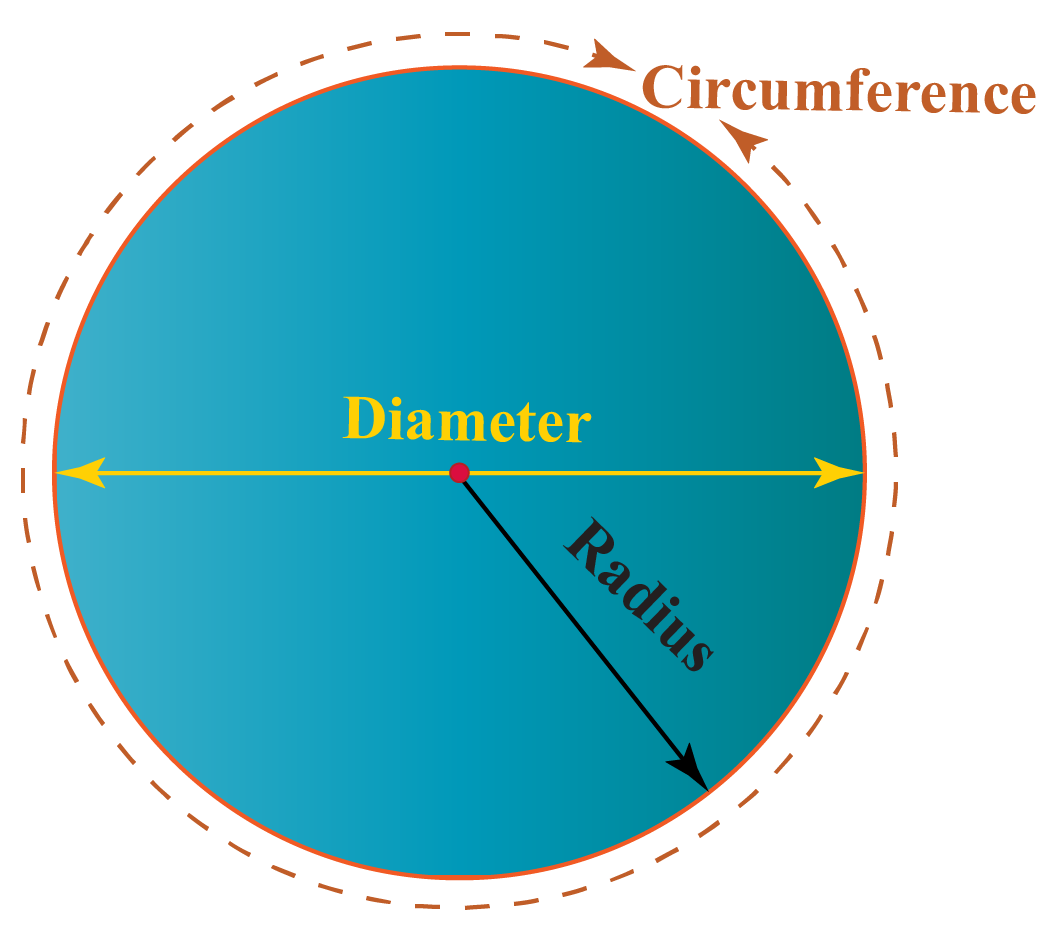Radius is the distance from the centre of a circle to the boundary of the circle.

### Diameter

Diameter is a straight line passing through the centre that connects two points on the boundary of the circle.

### Pi

Pi is a special mathematical constant with a value approximated to 3.14159 or $$\pi = \frac{22}{7}$$.

The value of $$\pi = \frac{22}{7}$$ is used in various formulas.

It is the ratio of circumference to diameter, where $$C = \pi D$$

## Formula for the Circumference of a Circle

The circumference of a circle can be calculated using the radius  '$$r$$' of the circle and the value of $$\pi$$.

 Circumference of a circle $$= 2\pi r$$

While using this formula, if we do not have the value of the radius, we can find it using the diameter.

That is, if the diameter is known, it can be divided by 2 to obtain the value of the radius because:

 Diameter of a circle $$= 2 \times r$$

Another way to calculate the circumference of a circle is by using the formula:

 Circumference $$= \pi \times Diameter$$

If we need to calculate the radius or diameter, when the circumference of a circle is given, we use the formula:

 \begin{align}Radius = \frac{Circumference}{2\pi }\end{align}

Help your child score higher with Cuemath’s proprietary FREE Diagnostic Test. Get access to detailed reports, customized learning plans, and a FREE counseling session. Attempt the test now.

## Solved Examples

 Example 1

A garland woven with flowers needs to be stuck around a circular ring to decorate it.

How long should the garland be so as to cover a ring of radius 28 cm?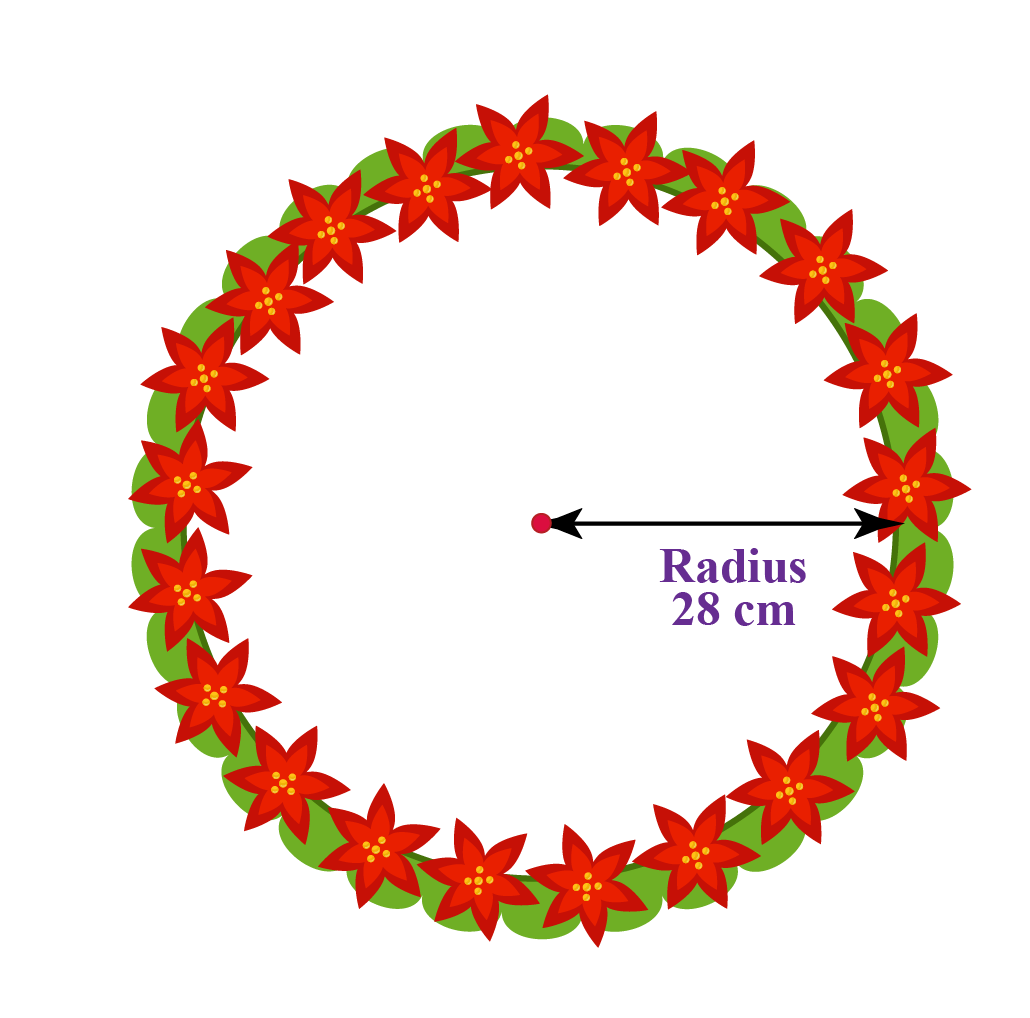Solution:

Given, Radius of the ring = 28 cm

To find the length of the garland, we have to find the circumference of the ring.

Circumference of a circle is calculated using the formula

\begin{align} &= 2\pi r \\ & = 2 \times \left(\frac{22}{7}\right) \times \left({28}\right) \\ & = \text{176} \text{ cm} \end{align}

 $$\therefore$$ Length of the garland $$= 176 \text { cm}$$
 Example 2

The circumference of a wheel is 440 cm.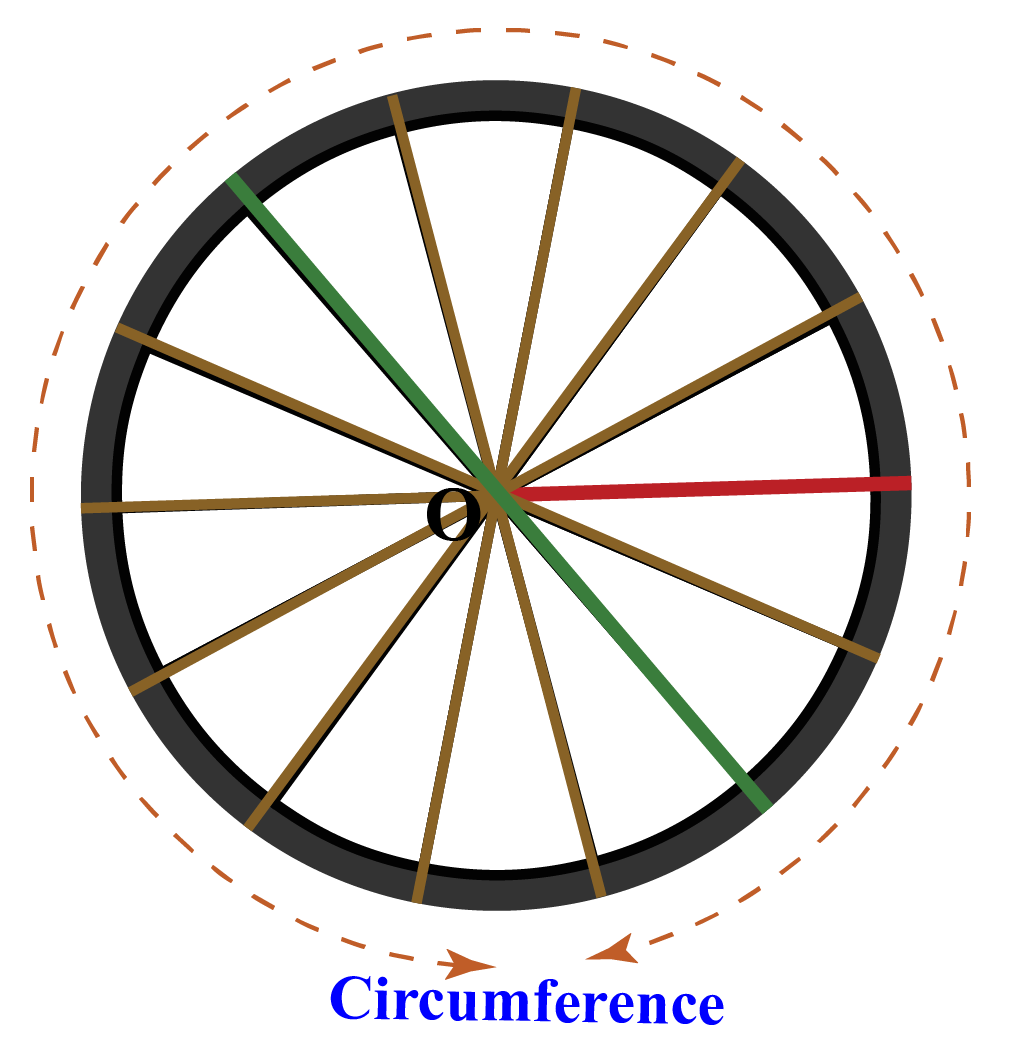Solution:

Given, Circumference of the wheel = 440 cm

Circumference of a circle $$= 2\pi r$$

Let the radius of the circle be R.

\begin{align} 440 &=2\pi R \\ 440& = 2 \times \left(\frac{22}{7}\right) \times \left({R}\right) \\ R& = \text{} \text{ 70 cm} \end{align}

Diameter $$= 2\text{r}$$

Diameter $$= 2\times {70}$$

 $$\therefore$$ Radius is 70 cm; Diameter is 140 cm
 Example 3

The perimeter of a rectangular wire is 264 m.

The same wire is bent into the shape of a circle.

Find the radius of the circle formed.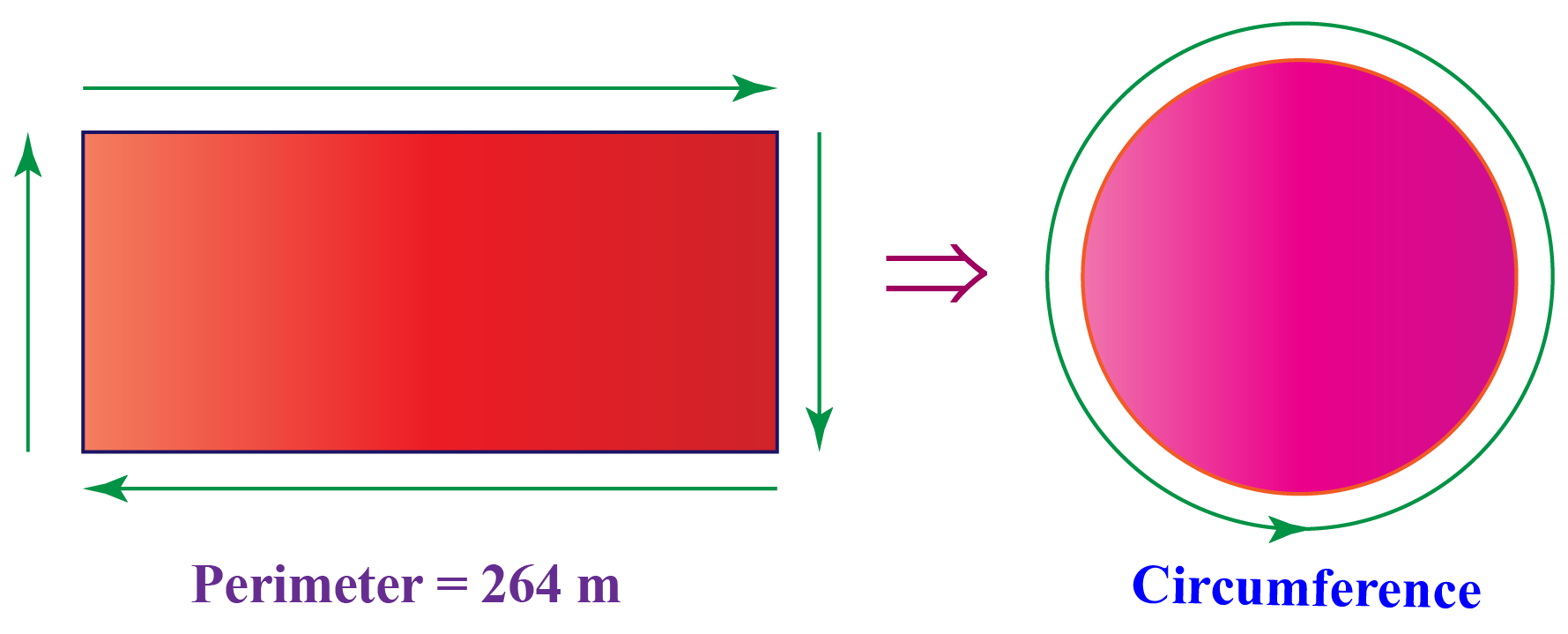Solution:

We know that, perimeter of the rectangle = Total length of the wire

Length of the wire used = Circumference of the circle formed

Hence, Circumference of the circle formed = 264 m

Circumference of a circle $$=2 \pi r$$

\begin{align} 2 \pi r &= 264 \text { m} \\ r&=42 \text { m} \end{align}

 $$\therefore$$ Radius is 42 m
 Example 4

Tom runs three rounds at a circular stadium field every day before starting his cricket practice.

The diameter of the field is 28 m.

How much does he run around the field every day?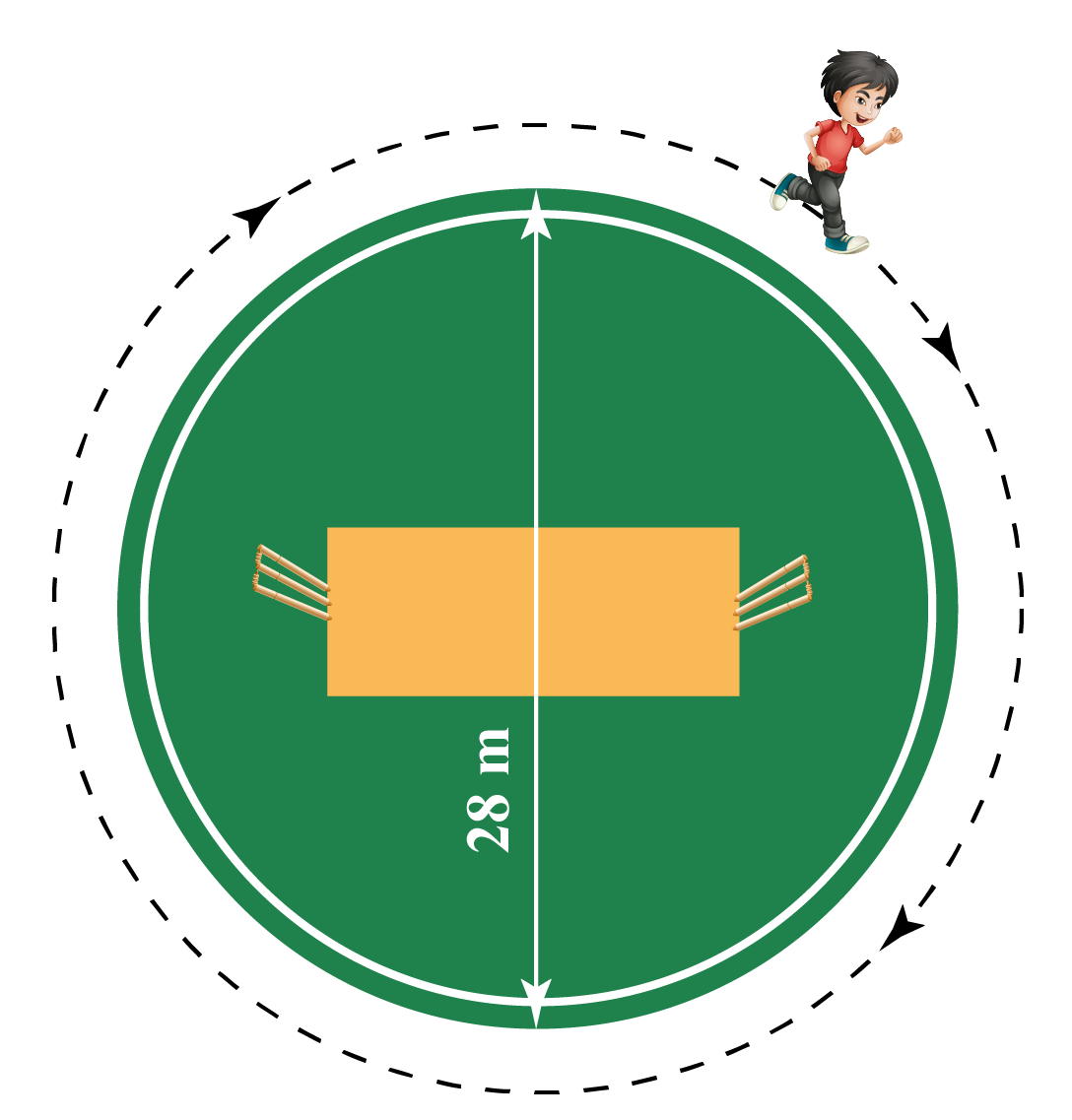Solution:

Since Tom runs around the circular field, we need to find the circumference of the field.

Given: $$Diameter = 28 \,\,m$$

\begin{align}Radius = \frac{28}{2}= 14 \, m\end{align}; because $$Diameter = 2r$$

Circumference of a Circle $$=2 \pi r$$

\begin{align} &= 2\pi r \\ & = 2 \times \left(\frac{22}{7}\right) \times \left({14}\right) \\ & = \text{88} \text{ m} \end{align}

Hence, the circumference of the field is 88 m.

We know that Tom runs around the field thrice.

Hence, we multiply the circumference by 3

$3 \times 88 =264\:\text{m}$

 $$\therefore$$ Tom runs 264 metres.
 Example 5

Find the radius of cake A whose circumference is 5 times that of the circumference of cake B with diameter 18 cm.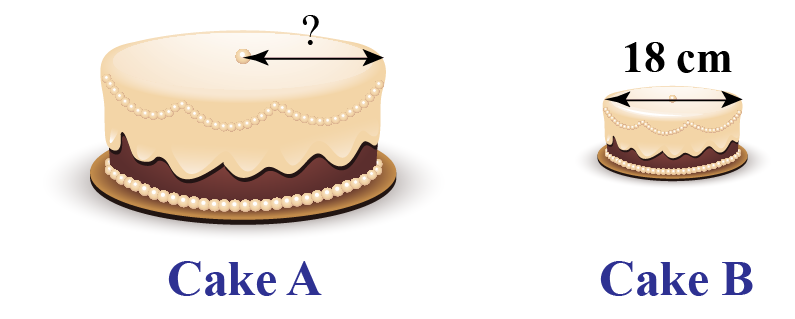Solution:

Diameter of cake B = 18 cm

Therefore, Radius of cake B = 9 cm as $$Diameter = 2r$$

Circumference of cake B $$=2 \pi r$$

\begin{align} &= 2\pi r \\ & = 2 \times \left(\frac{22}{7}\right) \times \left({9}\right) \\ & = \frac{396}{7} \end{align}

Since, the circumference of cake A is 5 times that of circumference of cake B, we get:

\begin{align} &= 5\times \frac{396}{7} \end{align}

Circumference of cake A \begin{align} &= \frac{1980}{7} \end{align}

Now, let's find the radius of cake A.

Let the radius of cake A be R cm.

Circumference of cake A:

\begin{align}\frac{1980}{7} & =2 \pi R \\ \frac{1980}{7} &= 2 \times \left(\frac{22}{7}\right) \times \left({R}\right) \end{align}

 $$\therefore$$ Radius of cake A is 45 cm.Challenging Question
1. The diameter of the given semi-circular slice of pizza is 28 cm. What is the perimeter of this slice of pizza?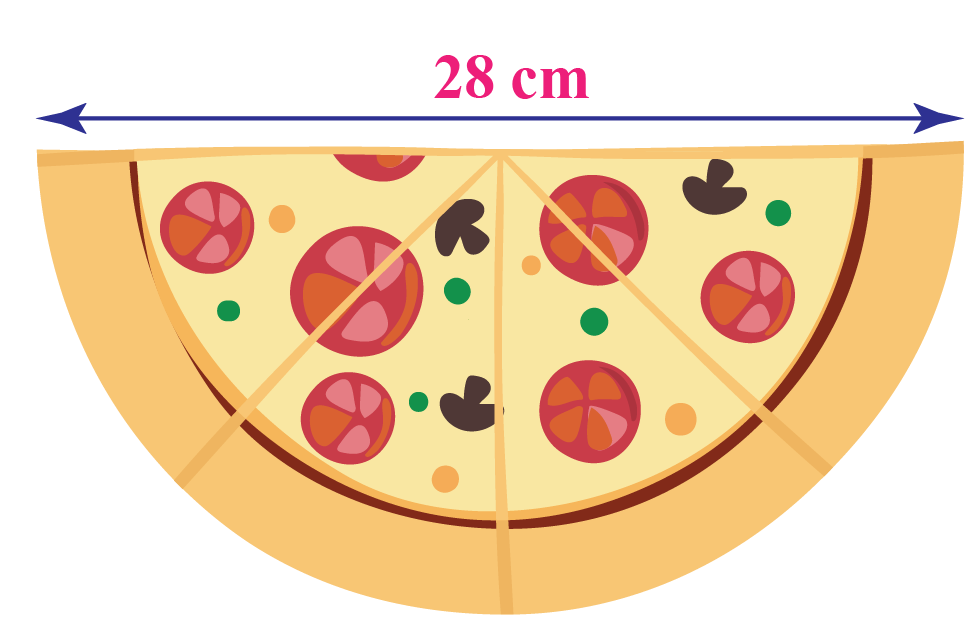Want to understand the “Why” behind the “What”? Explore Circumference of a Circle with our Math Experts in Cuemath’s LIVE, Personalised and Interactive Online Classes.

Make your kid a Math Expert, Book a FREE trial class today!

## Practice Questions

Try the Questions for Circumference of a Circle in the worksheet given below.

IMO (International Maths Olympiad) is a competitive exam in Mathematics conducted annually for school students. It encourages children to develop their math solving skills from a competition perspective.

## 1. What is the circumference of a circle?

The circumference of a circle is its boundary or the length of the complete arc of a circle.

## 2. How do you calculate the circumference of a circle?

The circumference of a circle is calculated with the help of a formula which needs the value of the radius and Pi.

Circumference of a circle $$= 2\pi r$$

## 3. How do you calculate the diameter from the circumference of a circle?

If we need to calculate the radius or diameter when the circumference of a circle is given, we use the formula: Circumference $$= \pi \times Diameter$$

More Important Topics
Numbers
Algebra
Geometry
Measurement
Money
Data
Trigonometry
Calculus